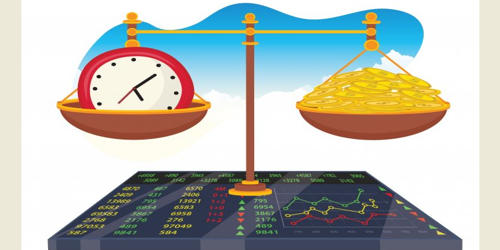Finance

# Volatility Arbitrage in FinanceArbitrage is the simultaneous purchase and sale (no risk) of the same asset from different markets. Volatility arbitrage refers to a type of statistical arbitrage strategy that is implemented in options trading. In finance, it is a type of statistical arbitrage that is implemented by trading a delta neutral portfolio of an option and its underlying. It is a trading strategy that attempts to profit from the difference between the forecasted future price-volatility of an asset, like a stock, and the implied volatility of options based on that asset. The objective is to take advantage of differences between the implied volatility of the option, and a forecast of future realized volatility of the option’s underlying. In volatility arbitrage, volatility rather than price is used as the unit of relative measure, i.e. traders attempt to buy volatility when it is low and sell volatility when it is high. This strategy can be implemented through a delta-neutral portfolio consisting of an option and its underlying asset. It is normally conducted in a delta-neutral portfolio comprising an option and its underlying asset.

Explanation

It generates profits from the difference between the implied volatility of options and the forecasted volatility of underlying assets. To an option trader engaging in volatility arbitrage, an option contract is a way to speculate in the volatility of the underlying rather than a directional bet on the underlying’s price. The values of options are impacted by the volatility of their underlying assets. If a trader buys options as part of a delta-neutral portfolio, he is said to be long volatility. If he sells options, he is said to be short volatility.

Higher volatility of the underlying asset leads to a higher value of the option. So long as the trading is done delta-neutral, buying an option is a bet that the underlying’s future realized volatility will be high while selling an option is a bet that future realized volatility will be low. Because of the put-call parity, it doesn’t matter if the options traded are calls or puts. Therefore, the different implied volatility of the option and forecasted volatility of the asset will generate a difference between the expected price and market price of the option. This is true because put-call parity posits a risk-neutral equivalence relationship between a call, a put, and some amount of the underlying. It is generally implemented in a delta-neutral portfolio with an option and its underlying asset. Therefore, being long a delta-hedged call results in the same returns as being long a delta-hedged put. Delta is a measure of the sensitivity of the derivative price to the change of its underlying asset price.